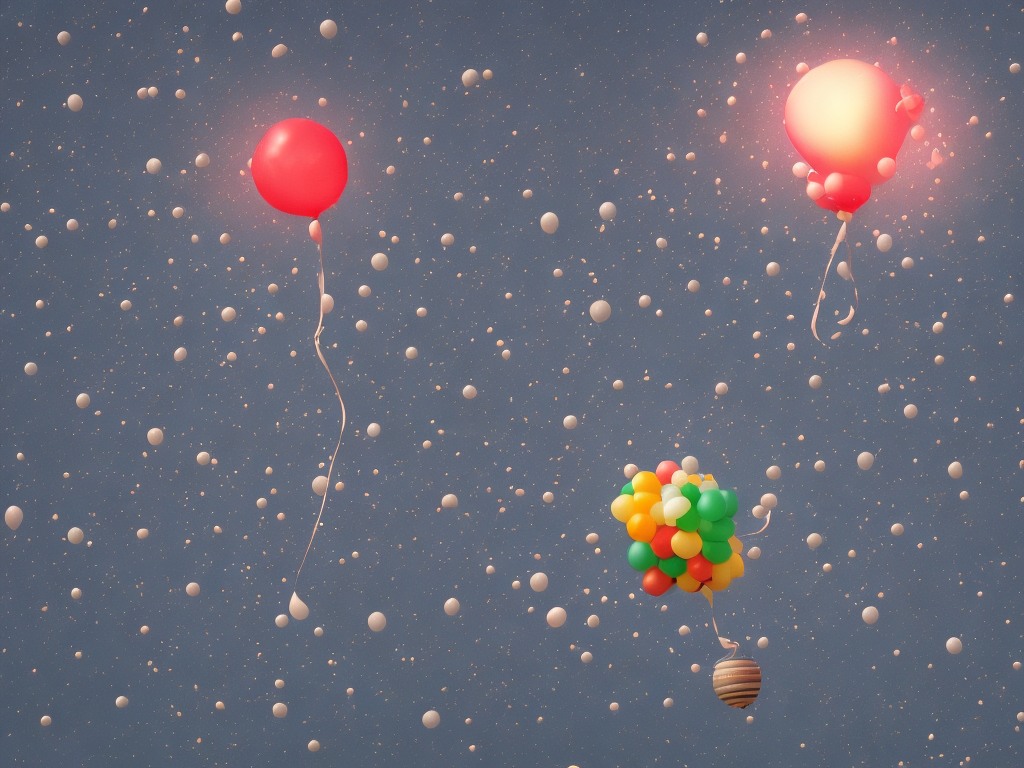# Difference Between Ideal Gas And Real Gas

tl;dr
The main difference between ideal and real gas is that ideal gas is an idealized concept with no volume or interactions between particles, while real gas has volume and interactions between particles.The main difference between ideal gas and real gas is that ideal gas is an idealized concept that assumes gas particles have no volume and no interactions between them, while real gas does not make these assumptions. Ideal gas is used to describe the behavior of gases under certain conditions, such as high temperatures and low pressures. Real gas, on the other hand, takes into account the volume of the gas particles, as well as the interactions between them.

Ideal gas is described by the ideal gas law, which states that the pressure of an ideal gas is directly proportional to its temperature and directly proportional to the number of moles of the gas. This law is also known as the equation of state. Real gas, however, does not follow this law exactly, as the interactions between the gas particles means that the gas will not behave as an ideal gas.

At low temperatures and high pressures, real gas particles tend to interact more strongly and the ideal gas law no longer holds. This is known as the deviation from ideal behavior. At high temperatures and low pressures, however, the interactions between the gas particles become weaker and the ideal gas law is more accurate.

In addition to the differences in behavior between ideal and real gas, the two gases also differ in terms of their physical properties. Ideal gas has no volume and no interactions between the particles, while real gas does have a volume and the particles interact with each other. This means that real gas is more compressible than ideal gas, which means that it can be compressed into a smaller volume than ideal gas.

In summary, the main difference between ideal gas and real gas is that ideal gas is an idealized concept that assumes gas particles have no volume and no interactions between them, while real gas does not make these assumptions. Real gas is more compressible than ideal gas and does not follow the ideal gas law exactly, due to the interactions between the gas particles.